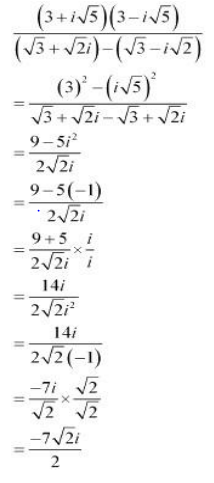# NCERT Solution Complex Number part -1

Question 1:
Express the given complex number in the form a + ib: (5i)(-3i/5)
Question 2:
Express the given complex number in the form a + ib:
i9 +i19
Question 3:
Express the given complex number in the form a + ib: i-39
Question 4:
Express the given complex number in the form a + ib: 3(7 + i7) + i(7 + i7)
Question 5:
Express the given complex number in the form a + ib: (1 – i) – (–1 + i6)
Question 6:
Express the given complex number in the form a + ib:
(1/5+2i/5)-(4+i5/2)
Question 7:
Express the given complex number in the form a + ib: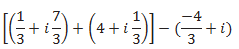Question 8
Express the given complex number in the form a + ib: (1 – i)4
Question 9:
Express the given complex number in the form a + ib: (1/3+3i)3
Question 10
Express the given complex number in the form a + ib: (-2-1i/3)3
Question 11:
Find the multiplicative inverse of the complex number 4 – 3i.
Question 12:
Find the multiplicative inverse of the complex number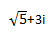Question 13
Find the multiplicative inverse of the complex number –i
Question 14
Express the following expression in the form of a + ib.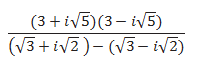Solution 1
(5i)(-3i/5)
Multiplying
=-(15/5)i2
Now we know that
i2=-1
so
=3
Solution 2
i9 +i19
=i2X4 +1 +i4X4 +3
=(i2)4 I + (i4)4 i3
Now i2=-1 so i4=1  and i3=-i
=i+(-i)
=i-i=0
Solution 3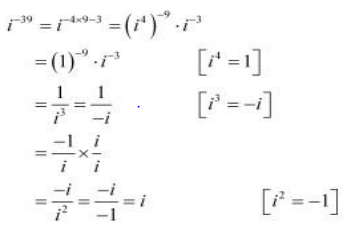Solution 4:
3(7 + i7) + i(7 + i7)
=21+21i+7i+7i2
Now i2=-1
So
=21+28i-7
=14+28i
Solution  5:
(1 – i) – (–1 + i6)
=2-7i
Solution 6:
(1/5+2i/5)-(4+i5/2)
=[(1/5)-4] +i[(2/5) –(5/2)]
=(-19/5) +(-21/10)i
Solution 7: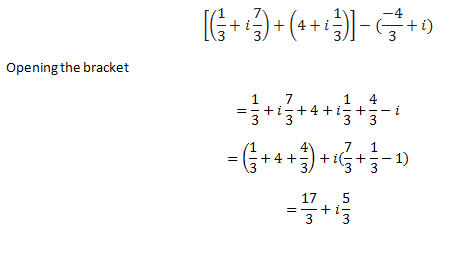Solution 8
(1 – i)4
Can be written as
=[(1-i)2]2
=(1+i2-2i)2
=(1-1-2i)2
=4i2=-4
Solution 9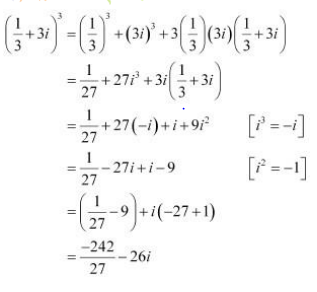Solution 10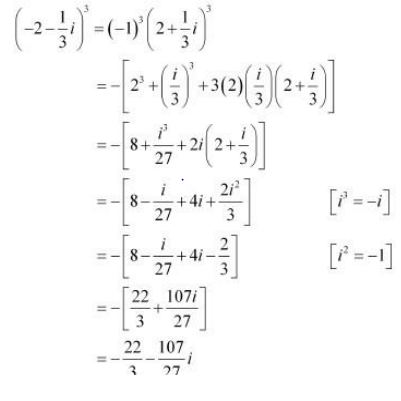Solution 11
Let z=4-3i
Conjugate is given by
=4+3i
Modulus is given by
|z|=5
Multiple inverse of any complex number z  is given by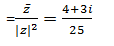Solution 12
Let z= √5+3i
Conjugate is given by
= √5-3i
Modulus is given by
|z|=5+9=14
Multiple inverse of any complex number z  is given by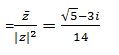Solution 13
Let z=-i
Conjugate is given by
=i
Modulus is given by
|z|=1
Multiple inverse of any complex number z  is given by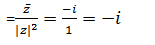Solution 14